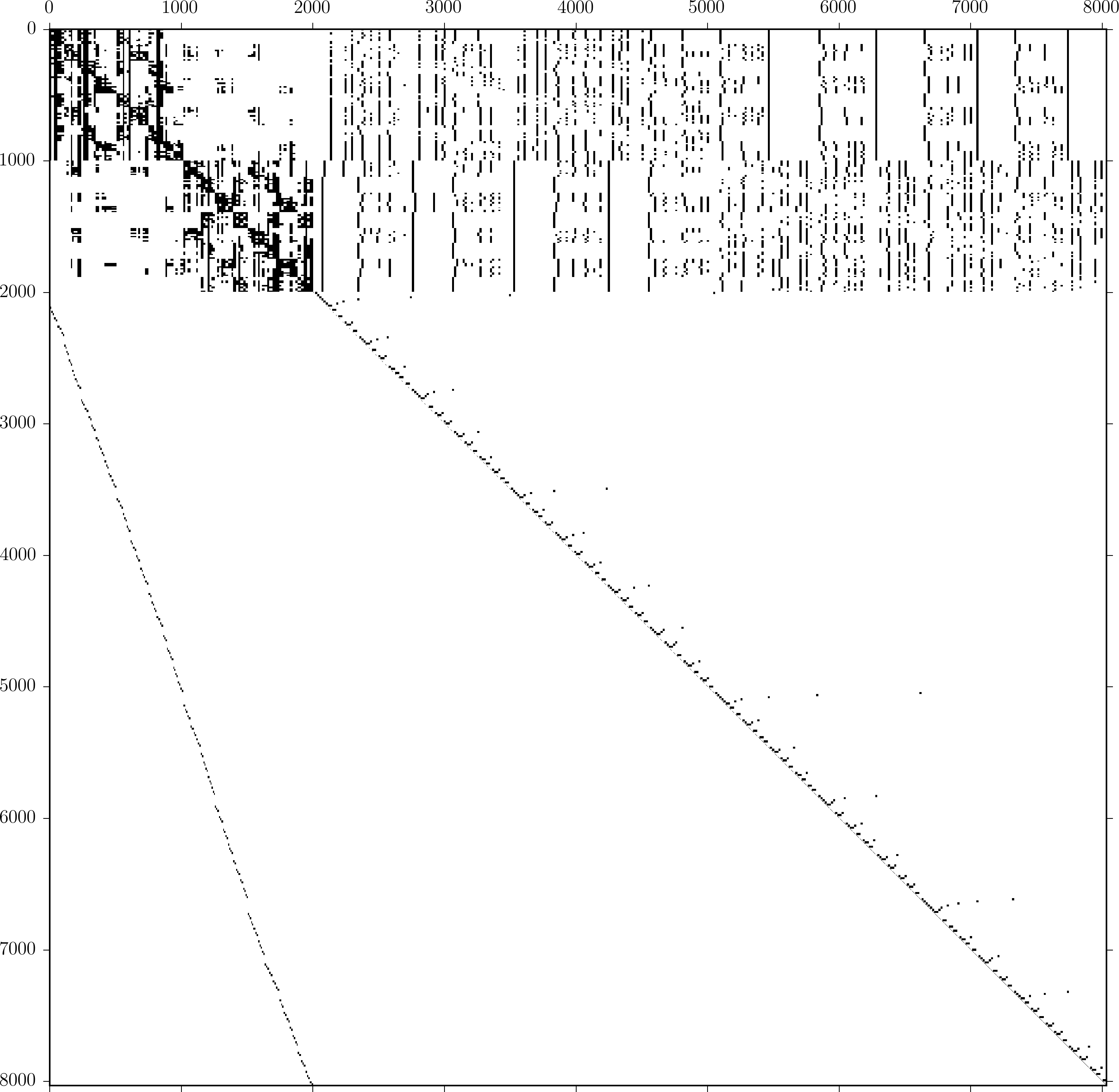## Sparse n-body matricesThis is a sparsity plot of a matrix representing an approximate n-body interaction. The black dots are non-zeros in the matrix. Imagine 2000 stars, each exerting some gravitational pull on each of the other stars. This gravitational interaction could be represented by a dense matrix:

$$A_{ij} = \frac{G m_i m_j}{|\mathbf{x}_i - \mathbf{x}_j|^2}$$

This works, but dense matrices can be slow when there are many stars. The cost of evaluating a matrix-vector product will scale like $O(n^2)$. Fortunately, the matrix can be represented in a different way if a little bit of error is okay. The interactions can be separated into those between nearby stars and the remaining interactions between stars that are far from each other. Groups of farfield interactions can be approximated. The result is a matrix that looks like what I’m showing above. The matrix has four distinct blocks. The upper left 2000x2000 block of the matrix represents the direct interaction that could not be approximated. The lower left and lower right represent the evaluation of the approximation coefficients. Both are super sparse! The upper right is the evaluation of the influence of the approximation coefficients on the points themselves. This part is less sparse.

Depending on exactly where the matrix coefficients are coming and how the approximation is done, this idea is called a tree-code, the fast multipole method, or a hierarchical matrix and results in an asymptotic complexity of either $O(n)$ or $O(n\log{n})$. The matrix in the figure is too small to show the impressive sparsification achievable with these methods. With a larger problem including 500,000 interacting points, I need <0.1% of the original number of matrix entries (250 billion vs 0.2 billion).

I’m using this same idea for elastic interactions in the Earth’s crust. The interaction kernel is different, but the idea is fundamentally the same. I’m using a specific variant of the fast multipole method called the kernel independent fast multipole method. This is a nice approach since the implementation is the same for each of the interaction kernels I deal with.